# [Java] [Processing × Java] How to use loop 2—Nested structure, coordinate transformation

![Screenshot 2020-07-03 16.03.55.png](https://qiita-image-store.s3.ap-northeast-1.amazonaws.com/0/654004/793268a0-97bb-be80-31ff-(7d61c0346c30.png)

# 0.for loop

## 0-0. for loop and coordinate transformation

In Processing, you can customize the “reference point when drawing a figure” and the “coordinate itself” at your convenience. At that time, the for loop is often used together. Below, I will write about coordinate transformation using a for loop.

###◯ Program to move the reference point of “Figure”

#### `for00.java`

``````
size(500,500);
background(255);
// Make it possible to draw a figure based on the center of the rectangle.
rectMode(CENTER);

Repeat //5 times (i = 0,1,2,3,4)
for(int i = 0;i <5;i++){
//Define an int type variable s that represents the size of the side of the rectangle.
int s = 400 -i * 80;

noFill();
stroke(115,237,201);
// Determine the thickness of the border
strokeWeight(3);
//Draw a rectangle with center at (width/2,height/2) and sides s.
rect(width/2,height/2,s,s);
}
``````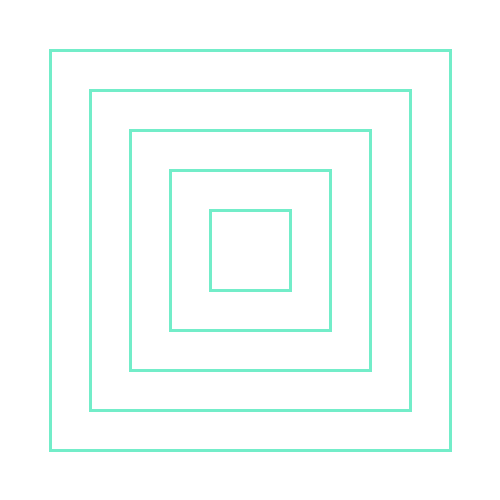Point: rectMode(CENTER) With this, you can draw a figure based on the center of the rectangle. (The default reference point is the upper left corner of the rectangle)

###◯ Program to shift the reference point of “coordinates” The following program uses translate() to shift the center of coordinates to (width/2,height/2). In other words, the coordinates of the center of the screen (Processing window) are now (0,0).

#### `for01.java`

``````
size(500,500);
background(255);
// Shift the coordinate reference point (origin) to the center of the screen (width/2, height/2)
translate(width/2,height/2);

//3 repeat
for(int i = 0;i <3;i++){
//TWO_PI/6 = 60 degrees, rotate every loop
rotate(TWO_PI/6);
noFill();
stroke(12,232,178);
// Since the center of the screen is (0,0)...
ellipse(0,0,150,375);
}
``````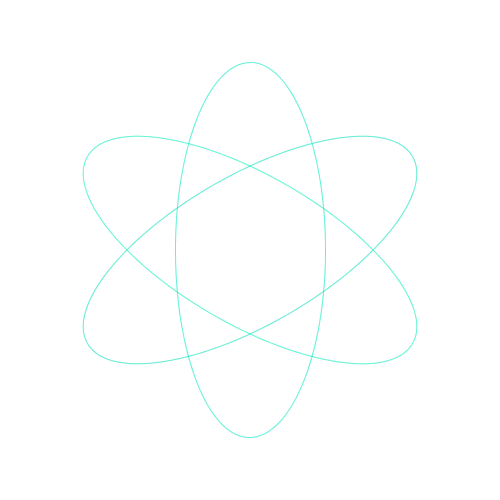Point: translate(); A function to shift the coordinate reference point. This time, the reference point was shifted to the center of the screen.

Point :rotate(); Rotate around the coordinate reference point. This time, the reference point is the center of the screen, so I rotated it around that axis. Arguments are specified using PI or TWO_PI.

Point :TWO_PI 2π = 360 degrees An angle is expressed by the length of a circular arc in a format called radian. The circumference of a unit circle with a radius of 1 is 2 * π = 2π. The arc 2π of the unit circle is the angle of one circle = 360 degrees.

## 0-1. for loop and nesting structure

###◯ Double nesting program

#### `for02.java`

``````
size(510,510);
background(255);
stroke(12,232,178);

// Repeat horizontal movement 5 times (i = 10,110,210,310,410)
for(int i = 10;i <width;i += 100){
// Repeat vertical movement 5 times (j = 10,110,210,310,410)
for(int j = 10;j <height;j += 100){
fill(12,232,178,255-i * 0.5);
rect(i,j,80,80);
}
}
``````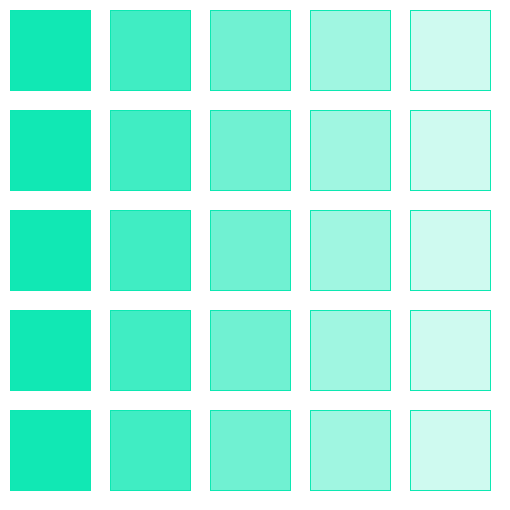Point: Program execution order

In the case of this program, it will be executed sequentially from the leftmost column (from the top).

That is ** When i = 10 ** ・J = 10: Execute ・J = 110: Execute ・J = 210: Execute ・J = 310: Execute ・J = 410: Execute When ◯i = 110 ・J = 10: Execute ・J = 110: Execute ・J = 210: Execute ・J = 310: Execute ・J = 410: Execute ** When i = 210 ** ::

###◯ Double nesting program (animation)

#### `draw.java`

``````
// I want to change the color, so prepare a variable c
int c = 0;

void setup(){
size(510,510);
}

// Gradually shift the variable c.
void draw(){
// Repeat horizontal movement 500 times (i = 0,1,2...508,509)
for(int i = 0;i <width;i ++){
// Repeat vertical movement 500 times (j = 0,1,2...508,509)
for(int j = 0;j <height;j++){
stroke(c,255-j/2,i/2);
// draw a 1 pixel dot
point(i,j);
}
}
c += 10;
// export colors
println(c);
// If the value of variable c exceeds 255
if(c> 255){
//set c to 0
c = 0;
}
}
// Runs only once when the mouse is pressed
void mousePressed(){
// Save the window when the mouse is pressed in png format
save("draw"+ c +".png");
// Output that the image has been saved
println("picture is saved.");
}
``````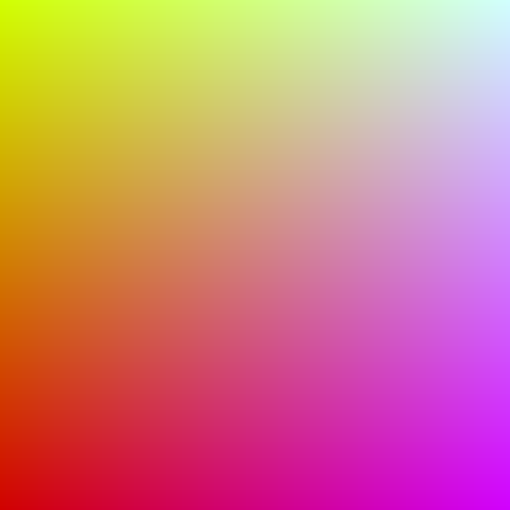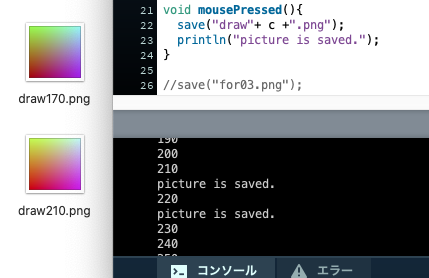Point :save() Images can be saved. Write the name and save format in “” such as save(“~.png”);. The saved image can be confirmed by [Open sketch folder] from [Sketch] of the menu bar.

###◯ Triple nesting program

#### `for03.java`

``````
size(510,510);
background(255);
noFill();
stroke(12,232,178);
// Make it possible to draw based on the center of the rectangle
rectMode(CENTER);

//5 repeats (i = 55,155,255,355,455)
for(int i = 55;i <width;i += 100){
Repeat //5 times (j = 55,155,255,355,455)
for(int j = 55;j <height;j += 100){
By repeating /4 times (s = 10,30,50,70), one square section is completed.
for(int s = 10;s <90;s += 20){
//Draw a square with side size s at coordinates (i,j)
rect(i,j,s,s);
}
}
}
``````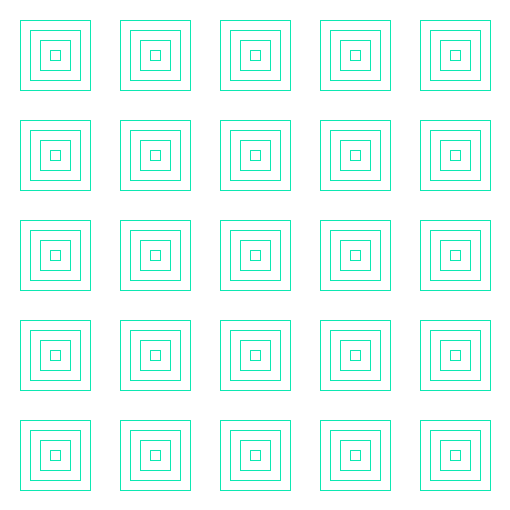Point: A mass of 4 squares ** like Matryoshka with different sizes

#### `.java`

``````
for(int s = 10;s <90;s += 20){
rect(,,s,s);
}
``````

Part of.

Put these chunks side by side (same as double loop).